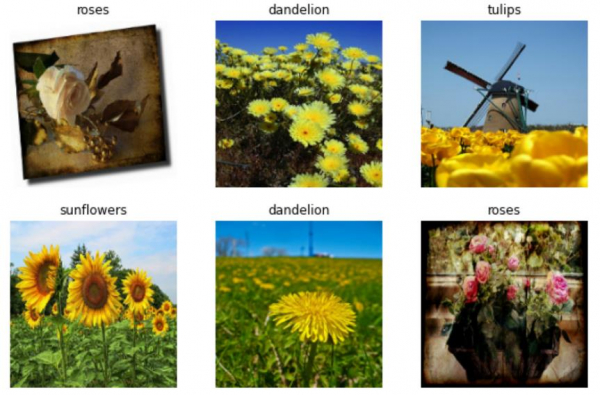# How can Tensorflow be used to visualize the flower dataset using Python?

The flower dataset can be visualized with the help of the ‘matplotlib’ library. The ‘imshow’ method is used to display the image on the console. The entire dataset is iterated over, and only the first few images are displayed.

We will be using the flowers dataset, which contains images of several thousands of flowers. It contains 5 sub-directories, and there is one sub-directory for every class.

We are using the Google Colaboratory to run the below code. Google Colab or Colaboratory helps run Python code over the browser and requires zero configuration and free access to GPUs (Graphical Processing Units). Colaboratory has been built on top of Jupyter Notebook.

import matplotlib.pyplot as plt

print("Visualizing the flower dataset")
plt.figure(figsize=(10, 10))
for images, labels in train_ds.take(1):
for i in range(6):
ax = plt.subplot(3, 3, i + 1)
plt.imshow(images[i].numpy().astype("uint8"))
plt.title(class_names[labels[i]])
plt.axis("off")

print("Iterating over dataset")
print("Retrieving batches of images")
for image_batch, labels_batch in train_ds:
print(image_batch.shape)
print(labels_batch.shape)
break

## Output

Visualizing the flower dataset
Iterating over dataset
Retrieving batches of images
(32, 180, 180, 3)
(32,)## Explanation

• The flower dataset is visualized using the matplotlib library.
• The first 6 images are iterated over and are displayed on the console.
• Again, the data set is iterated over, and the dimensions of the images are displayed on the console.